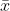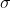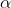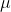##### Question

In: Statistics and Probability

# Construct a 95?% confidence interval to estimate the population mean using the following data. What assumptions...

Construct a 95?% confidence interval to estimate the population mean using the following data. What assumptions need to be made to construct this? interval?

 x?=93 ?=15 n=17

With 95?% ?confidence, when n=17 the population mean is between the lower limit of __ and the upper limit of __

## Solutions

##### Expert Solution

Solution :

Given that,= 93= 15

n = 17

At 95% confidence level the z is ,= 1 - 95% = 1 - 0.95 = 0.05/ 2 = 0.05 / 2 = 0.025

Z/2 = Z0.025 = 1.96

Margin of error = E = Z/2* (/n)

=1.96 * (15 / 17)

= 7.1305

At 99% confidence interval estimate of the population mean is,- E <<+ E

93-7.1305 << 93+7.1305

85.8695<< 100.1305

With 95?% ?confidence, when n=17 the population mean is between the lower limit of 85.8695 and the upper limit of 100.1305

## Related Solutions

##### Construct a 95% confidence interval to estimate the population mean using the following data. What assumptions...
Construct a 95% confidence interval to estimate the population mean using the following data. What assumptions need to be made to construct this​ interval? x(over bar x)= 83   s=19    n=16 What assumptions need to be made to construct this​ interval? A. The population is skewed to one side. B. The sample size is less than 30. C. The population mean will be in the confidence interval. D. The population must be normally distributed. With 95​% ​confidence, when n=16 the population...
##### Construct a 95% confidence interval to estimate the population mean using the data below. What assumptions need to be made about this population?
Construct a 95% confidence interval to estimate the population mean using the data below. What assumptions need to be made about this population? x̄ = 33 s= 9.9 n=27 The 95% confidence interval for the population mean is from a lower limit of _______  to an upper limit of _______ .
##### Construct a 90​% confidence interval to estimate the population mean using the accompanying data. What assumptions...
Construct a 90​% confidence interval to estimate the population mean using the accompanying data. What assumptions need to be made to construct this​ interval? x=59 σ=15 n=17 What assumptions need to be made to construct this​ interval? A. The population is skewed to one side. B. The sample size is less than 30. C. The population must be normally distributed. D. The population mean will be in the confidence interval. With 90​% ​confidence, when n=17​, the population mean is between...
##### Construct a 99​% confidence interval to estimate the population mean using the data below. What assumptions...
Construct a 99​% confidence interval to estimate the population mean using the data below. What assumptions need to be made about this​ population? x overbar =32 s=9.1 n=28
##### Construct a 95​% confidence interval to estimate the population mean using the data below. x̅= 46...
Construct a 95​% confidence interval to estimate the population mean using the data below. x̅= 46 σ=12 n= 41 With 95​% confidence, when n=41 the population mean is between a lower limit of ___ and upper limit of ___
##### Construct a? 95% confidence interval to estimate the population proportion using the data below. x =...
Construct a? 95% confidence interval to estimate the population proportion using the data below. x = 29 n = 90 N = 500 The? 95% confidence interval for the population proportion is? (_,_).
##### Construct a​ 95% confidence interval to estimate the population proportion using the data below.     x equals...
Construct a​ 95% confidence interval to estimate the population proportion using the data below.     x equals 27 n equals 75 N equals 500 The​ 95% confidence interval for the population proportion is (____,____)
##### Construct a​ 95% confidence interval to estimate the population proportion using the data below.     x equals...
Construct a​ 95% confidence interval to estimate the population proportion using the data below.     x equals 23, n equals 80, N equals 500 The​ 95% confidence interval for the population proportion is left parenthesis nothing comma nothing right parenthesis . ​(Round to three decimal places as​ needed.)
##### Construct a 95% Confidence Interval to estimate the population mean/proportion in the claim below. What can...
Construct a 95% Confidence Interval to estimate the population mean/proportion in the claim below. What can you conclude from this result regarding the claim? Claim: Is the proportion of people using public transportation is greater than private vehicles? Data: Ways to travel to work (100 people surveyed) Method Number Public Transportation 46 Private Vehicle 44 Uber, Lyft 10 Total 100
##### Refer to the accompanying data set and construct a 95​% confidence interval estimate of the mean...
Refer to the accompanying data set and construct a 95​% confidence interval estimate of the mean pulse rate of adult​ females; then do the same for adult males. Compare the results. Males : 86. 76. 49. 61. 55. 63. 53. 73. 53. 61. 74. 59. 63. 76. 85. 68. 63. 94. 41. 84. 74. 64. 69. 72. 55. 68. 58. 78. 69. 66. 64. 94. 56. 66. 60. 57. 68. 72. 87. 60. Females: 81. 91. 60. 68. 57. 81....